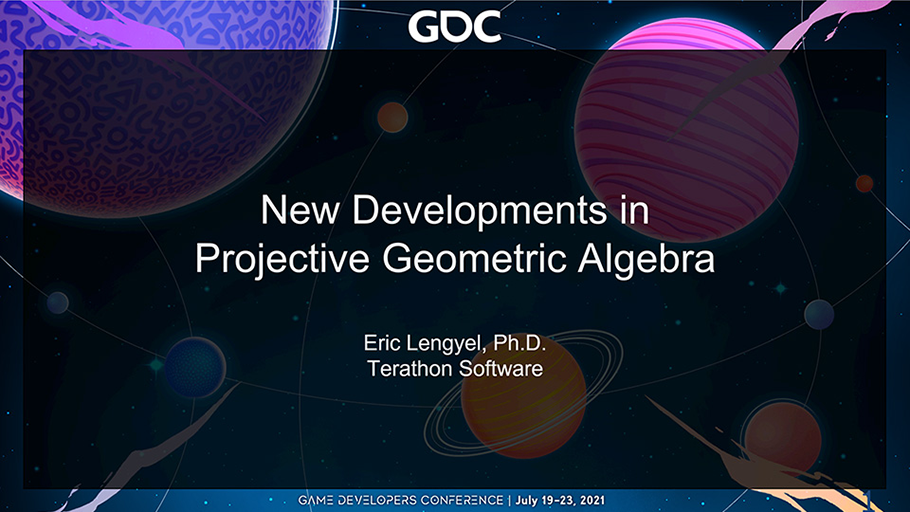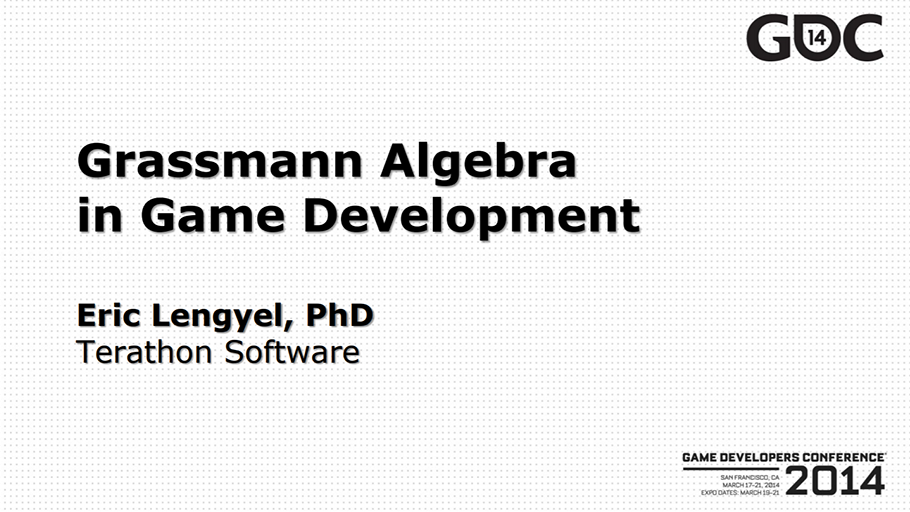# Projective Geometric Algebra

﻿

Projective Geometric Algebra

Projective geometric algebra (PGA) refers to a class of mathematical models in which geometric objects, operations for combining them in various ways, and
transformations that can be applied to them are all part of a single algebraic structure that is a specific type of Clifford algebra. This website deals with
two types of algebras called rigid geometric algebra and conformal geometric algebra, named for the types of transformations they contain.
Projective geometric algebra a natural fit for areas of computer science, especially computer graphics and robotics, that routinely use the mathematical concepts
that PGA encompasses.

The rigid geometric algebra (RGA) adds one dimension to ordinary space and incorporates representations for Euclidean points, lines, and planes as well as operations
for performing rotations, reflections, and translations. It completely subsumes conventional models that include homogeneous coordinates, Plücker coordinates,
quaternions, and screw theory (which makes use of dual quaternions). The conformal geometric algebra (CGA) adds two dimensions to ordinary space and includes everything
in the rigid geometric algebra. It also adds representations for round objects like circles and spheres as well as dilations, inversions, and other conformal transformations.
Both types of algebra contain operations for easily joining lower-dimensional geometries to form higher-dimensional geometries, for calculating intersections among all of
the geometries, and for projecting one kind of geometry onto another.

This page is a central resource containing all of the work on the subject of geometric algebra by Dr. Eric Lengyel.
A large repository of mathematical reference materials can be found on the following two wikis:

A C++ library that implements much of this math is available under the MIT license on GitHub.

The 36×24 inch reference posters below contain a huge amount of information, including new research from 2020–2023. Click on the images for PDF versions.
High-quality prints of these posters are available on Amazon at the links below each image.This website is an official silver sponsor of the symbols ⟑ and ⟇, which have Unicode values U+27D1 and U+27C7. These symbols denote the geometric product and geometric antiproduct in projective geometric algebra.
 New Developments in Projective Geometric Algebra These are the slides from the talk about PGA at the Game Developers Conference 2021. Download slides as PDFGrassmann Algebra in Game Development These are the slides from the talks about Grassmann algebra given at the Game Developers Conference in 2012 and 2014. Download slides as PDF

This site uses Akismet to reduce spam. Learn how your comment data is processed.